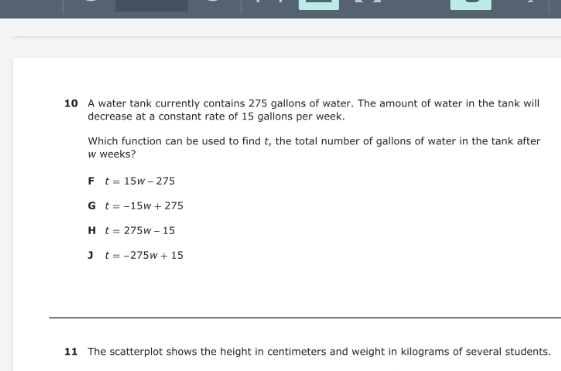### ¿Todavía tienes preguntas de matemáticas?

Pregunte a nuestros tutores expertos
Algebra
Pregunta$$1 0$$ A water tank currently contains $$275$$ gallons of water. The amount of water in the tank will decrease at a constant rate of $$15$$ gallons per week.

Which function can be used to find $$t$$ , the total number of gallons of water in the tank after

$$w$$ weeks?

$$t = 15 w - 275$$

$$t = - 15 w + 275$$

$$t = 275 w - 15$$

$$J t = - 275 w + 15$$